# 4 3 Balancing Chemical Equations Chemical reactions result

• Slides: 6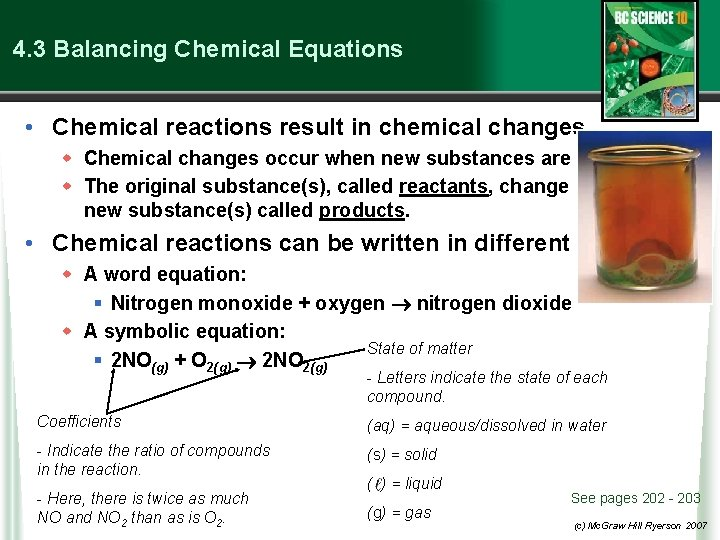4. 3 Balancing Chemical Equations • Chemical reactions result in chemical changes. w Chemical changes occur when new substances are created. w The original substance(s), called reactants, change into new substance(s) called products. • Chemical reactions can be written in different ways. w A word equation: § Nitrogen monoxide + oxygen nitrogen dioxide w A symbolic equation: State of matter § 2 NO(g) + O 2(g) 2 NO 2(g) - Letters indicate the state of each compound. Coefficients (aq) = aqueous/dissolved in water - Indicate the ratio of compounds in the reaction. (s) = solid - Here, there is twice as much NO and NO 2 than as is O 2. ( ) = liquid (g) = gas See pages 202 - 203 (c) Mc. Graw Hill Ryerson 2007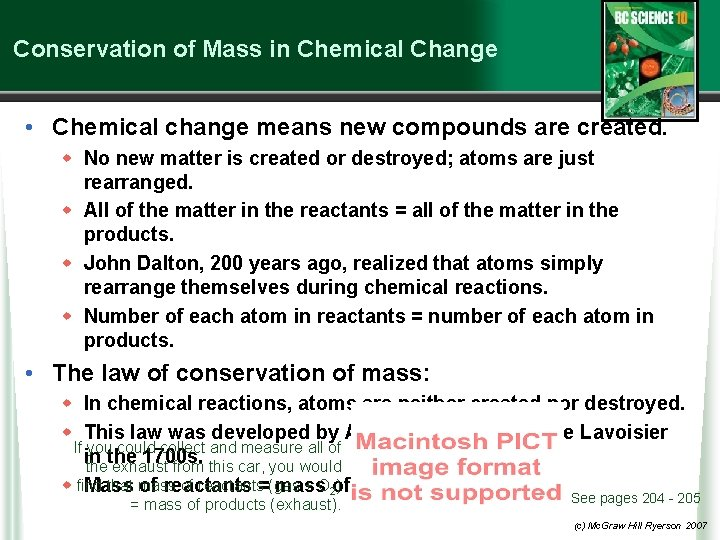Conservation of Mass in Chemical Change • Chemical change means new compounds are created. w No new matter is created or destroyed; atoms are just rearranged. w All of the matter in the reactants = all of the matter in the products. w John Dalton, 200 years ago, realized that atoms simply rearrange themselves during chemical reactions. w Number of each atom in reactants = number of each atom in products. • The law of conservation of mass: w In chemical reactions, atoms are neither created nor destroyed. w This law was developed by Antoine and Marie-Anne Lavoisier If you could collect and measure all of in the 1700 s. the exhaust from this car, you would that mass of reactants=(gas + O 2 of ) products w find Mass of reactants mass = mass of products (exhaust). See pages 204 - 205 (c) Mc. Graw Hill Ryerson 2007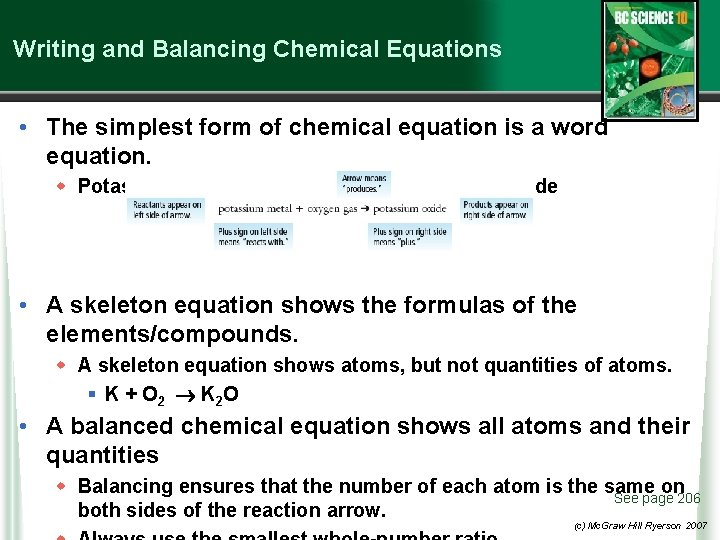Writing and Balancing Chemical Equations • The simplest form of chemical equation is a word equation. w Potassium metal + oxygen gas potassium oxide • A skeleton equation shows the formulas of the elements/compounds. w A skeleton equation shows atoms, but not quantities of atoms. § K + O 2 K 2 O • A balanced chemical equation shows all atoms and their quantities w Balancing ensures that the number of each atom is the same on See page 206 both sides of the reaction arrow. (c) Mc. Graw Hill Ryerson 2007Counting Atoms to Balance an Equation • Using the law of conservation of mass, we can count atoms to balance the number of atoms in chemical equations. w Word equation: methane + oxygen water + carbon dioxide w Skeleton equation: CH 4 + O 2 H 2 O + CO 2 § To balance the compounds, take note of how many atoms of each element occur on each side of the The same number reaction arrow. of atoms must be on each side. CH 4 + O 2 H 2 O + CO 2 1 carbon, 4 hydrogen, 2 oxygen 1 carbon, 2 hydrogen, 3 oxygen w To balance, attempt to find values that equate atoms on both See Page 207 sides (c) Mc. Graw Hill Ryerson 2007 w Skeleton equation: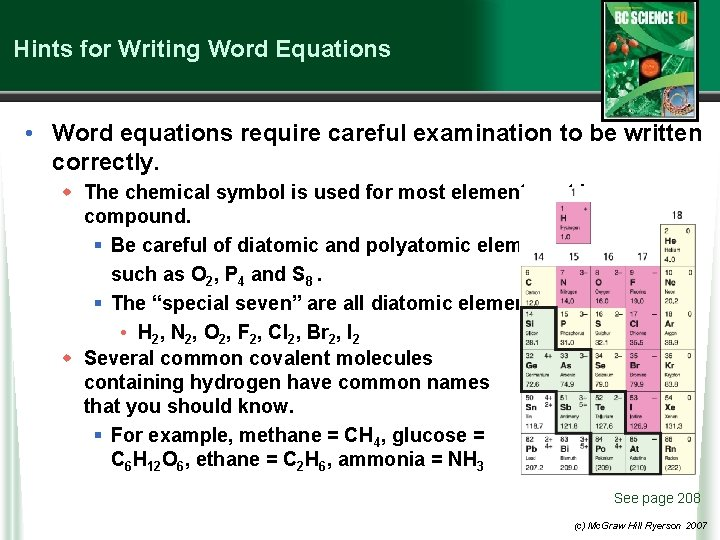Hints for Writing Word Equations • Word equations require careful examination to be written correctly. w The chemical symbol is used for most elements not in a compound. § Be careful of diatomic and polyatomic elements such as O 2, P 4 and S 8. § The “special seven” are all diatomic elements • H 2, N 2, O 2, F 2, Cl 2, Br 2, I 2 w Several common covalent molecules containing hydrogen have common names that you should know. § For example, methane = CH 4, glucose = C 6 H 12 O 6, ethane = C 2 H 6, ammonia = NH 3 See page 208 (c) Mc. Graw Hill Ryerson 2007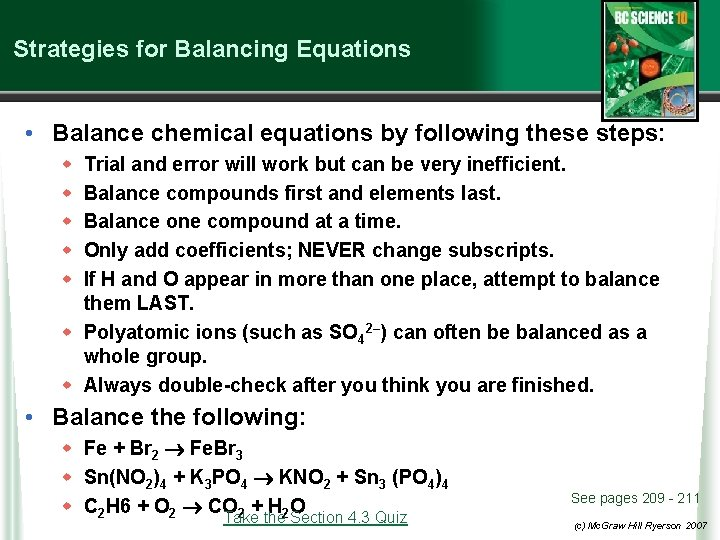Strategies for Balancing Equations • Balance chemical equations by following these steps: w w w Trial and error will work but can be very inefficient. Balance compounds first and elements last. Balance one compound at a time. Only add coefficients; NEVER change subscripts. If H and O appear in more than one place, attempt to balance them LAST. w Polyatomic ions (such as SO 42–) can often be balanced as a whole group. w Always double-check after you think you are finished. • Balance the following: w Fe + Br 2 Fe. Br 3 w Sn(NO 2)4 + K 3 PO 4 KNO 2 + Sn 3 (PO 4)4 w C 2 H 6 + O 2 CO 2 + H 2 O Take the Section 4. 3 Quiz See pages 209 - 211 (c) Mc. Graw Hill Ryerson 2007